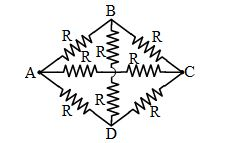﻿ Current Electricity, NCERT Physics Class 12 - MCQ Quiz by Dr. Mukesh Shrimali

# Current Electricity, Class 12 Physics MCQ Quiz Test

#### About the MCQ Pattern Practive Test Series

Find Chapter wise free online test of CBSE Class 12 Physics. The questions in these free online quizzes are important for CBSE Board Exam as well as Engineering and Medical Entrance Exams.
Find Chapter wise free online test for CBSE Class 12 Physics. The questions in these free online tests are important for CBSE Class 12 Physics Board Exam as well as Engineering and Medical Entrance Examinations. With the help of these tests or quizzes, one can quickly evaluate his or her preparation level for the subject.
You will find the correct answer of all the questions after you will submit the quiz. You will also get a report of your performance after you will submit the quiz.
Unlimited attempts possible to practice. The test contains 25 questions and there is no time limit to complete the quiz. The test is not official, it's just a nice way to see how much you know, or don't know, about Current Electricity.

#### How to Count Your Score

You will get 1 point for each correct answer. At the end of the Quiz, your total score will be displayed. Maximum score is 25 points.

Good Luck, Lets start the Quiz on Physics Class 12 - Current Electricity

MCQ 1:
In the adjoining network of resistors, each is of resistance r ohm, the equivalent resistance between points A and B is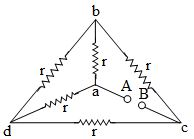MCQ 2:
In the following figure, the ratio of current in 3 Ω and 1 Ω resistances is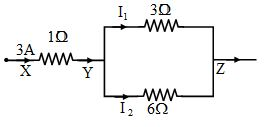MCQ 3:
The resultant resistance between the points A and B in the following diagram Fig. will be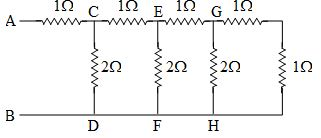MCQ 4:
Current in a conductor is due to

MCQ 5:
Specific resistance of a wire depends on the

MCQ 6:
The resistance of some substances become zero at very low temperature , then these substances are called

MCQ 7:
The resistance of wire is 20Ω. The wire is stretched to three times its length. Then the resistance will now be

MCQ 8:
When the temperature of a metallic conductor is increased its resistance

MCQ 9:
Ohm's law deals with the relation between

MCQ 10:
Net resistance between X and Y is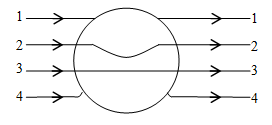MCQ 11:
Net resistance between X and Y is -MCQ 12:
The equivalent resistance between the terminal point P and Q is 4Ω in the given circuit, then find out the resistance of R in ohms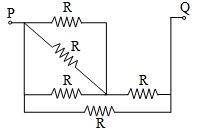MCQ 13:
For following circuit the value of total resistance between X and Y in ohm is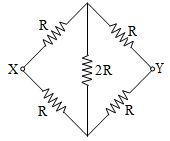MCQ 14:
When n identical resistances of value r each are connected in parallel, the equivalent resistance is x. The resultant resistance when they are connected in series is

MCQ 15:
The effective resistance (in Ω) between (B) and (C) of letter (A) , containing resistance as shown in fig. as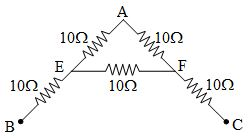MCQ 16:
Five identical resistance are connected as shown in fig. The equivalent resistance between point (A) and (B) is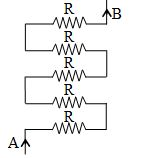MCQ 17:
In the following circuit the resultant emf (electromotive force) between AB is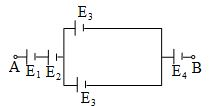MCQ 18:
The current (I) and voltage (V) graphs for a given metallic wire at two different temperature (T1) and (T2) are shown in fig. It is concluded that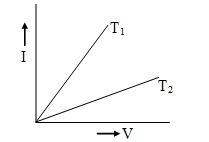MCQ 19:
In fig the equivalent resistance between points (x) and (y)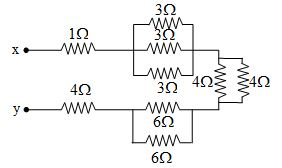MCQ 20:
A 3 volt battery with negligible internal resistance is connected in a circuit as shown in the figure. The current I in the circuit will be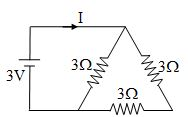MCQ 21:
The current I drawn from the 5 volt source will be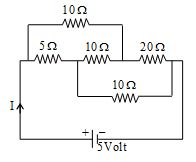MCQ 22:
The Kirchhoffs first law (Σi = 0) and second law (ΣiR = ΣE), where the symbols have usual meanings, are respectively based on

MCQ 23:
The equivalent resistance between points A and B of the circuit given below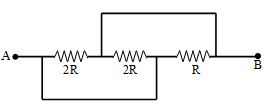MCQ 24:
A cylindrical wire is stretched to increase its length by 10%. The percentage increase in the resistance of the wire will be

MCQ 25:
The equivalent resistance between A and C is